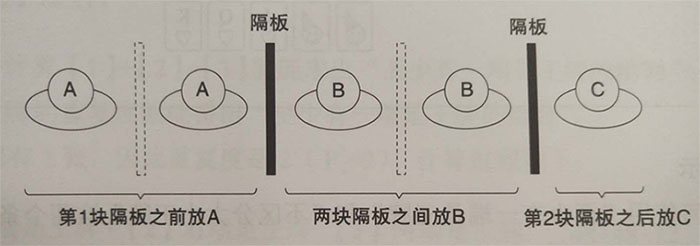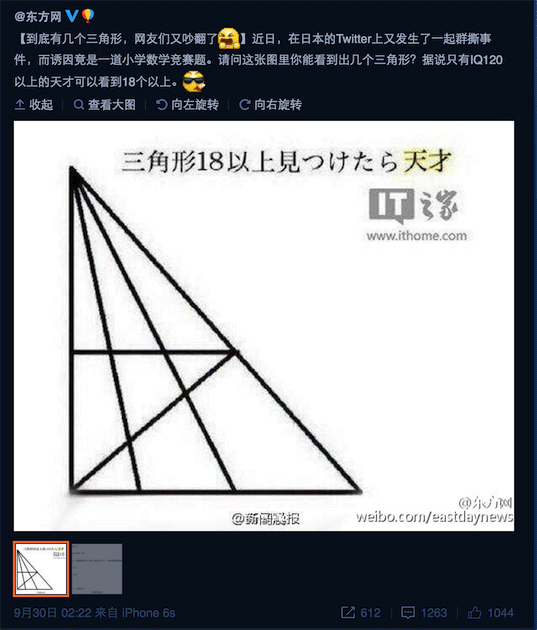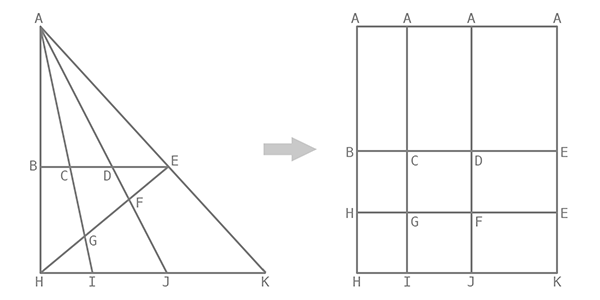• A、B、C 这三种药丸每种至少有1粒
• 不考虑药丸的顺序
• 同种药丸每粒都相同

### 排列组合

$P_n^k = C_n^k \times P_k^k = \frac{n!}{(n-k)!}$### 三角形问题